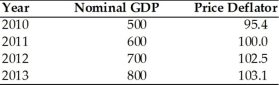• FAQ
• Contact/ Homework Answers / Economics / 21) Suppose that the following occurred in two countries during
Not my Question
Flag Content

# Question

21) Suppose that the following occurred in two countries during the past decade. Country A, real Gross Domestic Product (GDP) rose 40 percent and population rose 46 percent; Country B, real Gross Domestic Product (GDP) increased 80 percent and population increased 75 percent. Based on this information, which is true?

A) Both countries have experienced growth in per capita real Gross Domestic Product (GDP).

B) Neither country has experienced growth in per capita real Gross Domestic Product (GDP).

C) Chances for an improved standard of living are greater in Country A.

D) Only Country B has experienced growth in its per capita real Gross Domestic Product (GDP).22) Refer to the above table. Real GDP in 2010 is

A) 190.8.

B) 477.

C) 500.

D) 524.1.

23) Refer to the above table. Real GDP in 2012 is

A) 146.4.

B) 682.9.

C) 700.

D) 717.5.

24) Refer to the above table. Real GDP in 2013 is

A) 103.1.

B) 128.9.

C) 775.9.

D) 824.8.

25) Refer to the above table. All real GDP numbers are expressed in terms of the purchasing power of dollars in

A) 2010.

B) 2011.

C) 2012.

D) 2013.

26) When GDP and other national income accounts are measured in nominal values, then they

A) are measured in current market prices.

B) are measured in real values.

C) are not comparable because they are not in real terms.

D) should be converted into money values before comparing them over time.

27) Between two years, GDP at constant prices increased by 5 percent while GDP at current prices increased by 8 percent. Based on this information, the price level

A) increased by 3 percent.

B) increased by 13 percent.

C) decreased by 3 percent.

D) decreased by 13 percent.

28) The GDP deflator is equal to.

A) nominal GDP divided by real GDP.

B) nominal GDP multiplied by real GDP.

C) nominal GDP minus real GDP.

D) nominal GDP plus real GDP.

29) Which of the following represents how real GDP is calculated?

A) nominal GDP multiplied by the GDP deflator

B) nominal GDP divided by the population

C) nominal GDP divided by the PPI, the producer price index

D) nominal GDP divided by the GDP deflator

30) The nominal value of an economic variable is

A) the consumer price index.

B) the producer price.

C) expressed in terms of actual market prices at which goods are sold.

D) computed by taking the nominal value and dividing by the appropriate price index.

## Solution 5 (1 Ratings )

Solved
Economics 2 Months Ago 5 Views